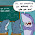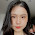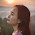## Sunday, July 31, 2016

### Faster Than SGD 1: Variance Reduction

SGD is well-known for large-scale optimization. In my mind, there are so-far two fundamentally different improvements since the original introduction of SGD: (1) variance reduction, and (2) acceleration. In this post I'd love to conduct a survey regarding (1), and I'd like to especially thank those ICML'16 participants who pushed me to write this post.

Consider the famous composite convex minimization problem
\begin{equation}\label{eqn:the-problem}
\min_{x\in \mathbb{R}^d} \Big\{ F(x) := f(x) + \psi(x) := \frac{1}{n}\sum_{i=1}^n f_i(x) + \psi(x) \Big\} \enspace, \tag{1}
\end{equation}
in which $f(x) = \frac{1}{n}\sum_{i=1}^n f_i(x)$ is a finite average of $n$ functions, and $\psi(x)$ is simple "proximal" function such as the $\ell_1$ or $\ell_2$ norm. In this finite-sum form, each function $f_i(x)$ usually represents the loss function with respect to the $i$-th data vector. Problem \ref{eqn:the-problem} arises in many places:
• convex classification and regression problems (e.g. Lasso, SVM, Logistic Regression) fall into \ref{eqn:the-problem}.
• some notable non-convex problems including PCA, SVD, CCA can be reduced to \ref{eqn:the-problem}.
• the neural net objective can be written in \ref{eqn:the-problem} as well although the function $F(x)$ becomes non-convex; in any case, methods solving convex versions of \ref{eqn:the-problem} sometimes do generalize to non-convex settings.

## Recall: Stochastic Gradient Descent (SGD)

To minimize objective $F(x)$, stochastic gradient methods iteratively perform the following update
$$x_{k+1} \gets \mathrm{argmin}_{y\in \mathbb{R}^d} \Big\{ \frac{1}{2 \eta } \|y-x_k\|_2^2 + \langle \tilde{\nabla}_k, y \rangle + \psi(y) \Big\} \enspace,$$
where $\eta$ is the step length and $\tilde{\nabla}_k$ is a random vector satisfying $\mathbb{E}[\tilde{\nabla}_k] = \nabla f(x_k)$ and is referred to as the gradient estimator. If the proximal function $\psi(y)$ equals zero, the update reduces to $x_{k+1} \gets x_k - \eta \tilde{\nabla}_k$.
A popular choice for the gradient estimator is $\tilde{\nabla}_k = \nabla f_i(x_k)$ for some random index $i \in [n]$ per iteration, and methods based on this choice are known as stochastic gradient descent (SGD). Since computing $\nabla f_i(x)$ is usually $n$ times faster than that of $\nabla f(x)$, SGD enjoys a low per-iteration cost as compared to full-gradient methods; however, SGD cannot converge at a rate faster than $1/\varepsilon$ even if $F(\cdot)$ is very nice.

## Key Idea: Variance Reduction Gives Faster SGD

The theory of variance reduction states that, SGD can converge much faster if one makes a better choice of the gradient estimator $\tilde{\nabla}_k$, so that its variance "reduces as $k$ increases". Of course, such a better choice must have (asymptotically) the same per-iteration cost as compared with SGD.

There are two fundamentally different ways to choose a better gradient estimator, the first one is known as SVRG, and the second one is known as SAGA (which is built on top of SAG). Both of them require each function $f_i(x)$ to be smooth, but such a requirement is not intrinsic and can be somehow removed.

• Choice 1: the SVRG estimator (my favorite)
Keep a snapshot vector $\tilde{x} = x_k$ every $m$ iterations (where $m$ is some parameter usually around $2n$), and compute the full gradient $\nabla f(\tilde{x})$ only for such snapshots. Then, set
$$\tilde{\nabla}_k := \nabla f_i (x_k) - \nabla f_i(\tilde{x}) + \nabla f(\tilde{x})$$
where $i$ is randomly chosen from $1,\dots,n$. The amortized cost of computing $\tilde{\nabla}_k$ is only 3/2 times bigger than SGD if $m=2n$ and if we store $\nabla f_i(\tilde{x})$ in memory.
• Choice 2: the SAGA estimator.
Store in memory $n$ vectors $\phi_1,\dots,\phi_n$ and set all of them to be zero at the beginning. Then, in each iteration $k$, set
$$\tilde{\nabla}_k := \nabla f_i(x_k) - \nabla f_i(\phi_i) + \frac{1}{n} \sum_{j=1}^n \nabla f_j(\phi_j)$$
where $i$ is randomly chosen from $1,\dots,n$. Then, very importantly, update $\phi_i \gets x_k$ for this $i$. If properly implemented, the per-iteration cost to compute $\tilde{\nabla}_k$ is the same as SGD.

## How Variance is Reduced?

Both choices of gradient estimators ensure that the variance of $\tilde{\nabla}_k$ approaches to zero as $k$ grows. In a rough sense, both of them ensure that $\mathbb{E}[\|\tilde{\nabla}_k - \nabla f(x_k)\|^2] \leq O(f(x_k) - f(x^*))$ so the variance decreases as we approach to the minimizer $x^*$. The proof of this is two-lined if $\psi(x)=0$ and requires a little more effort in the general setting, see for instance Lemma A.2 of this paper

Using this key observation one can prove that, if $F(x)$ is $\sigma$-strongly convex and if each $f_i(x)$ is $L$-smooth, then the "gradient complexity" (i.e., # of computations of $\nabla f_i (x)$) of variance-reduced SGD methods to minimize Problem \ref{eqn:the-problem} is only $O\big( \big(n + \frac{L}{\sigma} \big) \log \frac{1}{\varepsilon}\big)$. This is much faster than the original SGD method.

## Is Variance Reduction Significant?

Short answer: NO when first introduced, but becoming YES, YES and YES.

Arguably the original purpose of variance reduction is to make SGD run faster on convex classification / regression problems. However, variance-reduction methods cannot beat the slightly-earlier introduced coordinate-descent method SDCA, and performs worse than its accelerated variant AccSDCA (see for instance the comparison here).

Then why is variance reduction useful at all? The answer is on the generality of Problem \eqref{eqn:the-problem}. In all classification and regression problems, each $f_i(x)$ is of a restricted form $loss(\langle a_i, x\rangle; b_i)$ where $a_i$ is the $i$-th data vector and $b_i$ is its label. However, Problem \eqref{eqn:the-problem} is a much bigger class, and each function $f_i(x)$ can encode a complicated structure of the learning problem. In the extreme case, $f_i(x)$ could encode a neural network where $x$ characterize the weights of the connections (so becoming nonconvex). For such general problems, SDCA does not work at all.

In sum, variance reduction methods, although converging in the same speed as SDCA, applies more widely.

## The History of Variance Reduction

There are too many variance-reduction papers that even an expert sometime can't keep track of all of them. Below, let me point out some interesting papers that one should definitely cite:
• The first variance-reduction method is SAG.
However, SAG is not known to work in the full proximal setting and thus (in principle) does not apply to for instance Lasso or anything L1-regularized. I conjecture that SAG also works in the proximal setting, although some of my earlier experiments seem to suggest that SAG is outperformed by its gradient-unbiased version SAGA in the proximal setting.
• SAGA is a simple unbiased fix of SAG, and gives a much simpler proof than SAG. In my experiments, SAGA seems performing never worse than SAG.
• SVRG was actually discovered independently by two groups of authors, group 1 and group 2. Perhaps because there is no experiment, the first group's paper quickly got unnoticed (cited by 24) and the second one becomes very famous (cited by 200+). What a pity.
Because SAGA and SVRG are the popular choices, one may ask which one runs faster? My answer is
• It depends on the structure of the dataset: a corollary of this paper suggests that if the feature vectors are pairwisely close, then SVRG is better, and vice versa.
• Experiments seem to suggest that if all vectors are normalized to norm 1, then SVRG performs better.
• If the objective is not strongly convex (such as Lasso), then a simple modification of SVRG outperforms both SVRG and SAGA.
• Also, when $f_i(x)$ is a general function, SAGA requires $O(nd)$ memory storage which could be too large to load into memory; SVRG only needs $O(d)$.

## What's Next Beyond Variance Reduction?

There are many works that tried to extend SVRG to other settings. Most of them are no-so-interesting tweaks, but there are three fundamental extensions.
• Shalev-Shwartz first studied Problem \ref{eqn:the-problem} but each $f_i(x)$ is non-convex (although the summation $f(x)$ is convex). He showed that SVRG also works there, and this has been later better formalized and slightly improved. This class of problems has given rise to the fastest low-rank solvers (in theory) on SVD and related problems.
• Elad and I showed that SVRG also works for totally non-convex functions $F(x)$. This is independently discovered by another group of authors. They published at least two more papers on this problem too, one supporting proximal, and one proving SAGA's variant.
The above two improvements are regarding what will happen if we enlarge the class of Problem \ref{eqn:the-problem}. The next improvement is regarding the same Problem \ref{eqn:the-problem} but an even faster running time:

1.This comment has been removed by the author.

2.Great ~~~ Thanks a lot! Post more :- )

3.It's good if you upload articles frequently. Your article is very interesting.I apply your advice on daily situations. I wait for new articles. They have lots of knowledge and tips.
best autoketing, Shopify free shipping bar app, shipping bar app

4.A debt of gratitude is in order for the post.I'm full of the joys of spring when see your new posts.Your posts can help me more and more in the future. I'll unquestionably return. app autoketing, recent sales notification popup, sales pop master app

5.The site is very interesting, you made some compelling remarks and the matter is on point. The next occasion I read a weblog, Lets hope so it doesnt disappoint me as much as this.
autoketing app, facebook live chat help, facebook chat box

6.I feel good when reading your posts. Those are what I want to know. Thank you for introducing useful information to us
autoketing
email with love by autoketing

7.8.This is an unprecedented article, Given such a great deal of information in it, These kind of articles keeps the clients enthusiasm for the site, and continue sharing more ... positive conditions.
abcya3 for school motox3m3 games online gogy Games for boys

9.Free Crime city 3d 2 games
Games Flyordieio
The story of cinderella Games unblocked
I recently found many useful information in your website especially this blog page. Among the lots of comments on your articles. Thanks for sharing.

10.Thanks to your sharing, I can enrich my knowledge.It is very nice of you to share your understanding.Thank you so much. I usually wait for your pieces of writing. girl game Rapunzel Fashionista Busy Day Game Break The Cup Game Battle for the Galaxy

11.Your articles are very useful. Thanks to your sharing, I can enrich my knowledge.They are useful pieces of advice.
Tomb Runner free game Mommy Elsa Baby Caring play for free Ride The Bus Simulator funny game

12.This is a great inspiring article.I am pretty much pleased with your good work.You put really very helpful information. Keep it up. Keep blogging. Looking to reading your next post.
4 player sheep party games
Gunmaster onslaught games for kid
Colorzzle games to play

13.Delight maintain this approach awesome succeed and additionally Document wait for a great deal more on your stunning blog posts. I just want to let you know that I just check out your site and I find it very interesting and informative.
Fire truck crazy race free
Free unblock the ball
Games princess and adult princess

14.Hi Friend!
orlando escorts Have a great day!

15.Candy crush 422 Degree is fighting Against chocolate spawners and popcorns

Candy crush 422 degree is the most hard amount. It creates challenging in Chocolate spawners. In the event the chocolate spawners do not see the match gets very effortless. So this amount we consistently try how to remove chocolate spawners. This level also has significantly more problems. It's some popcorn. It will also remove challenges. Chocolate spawners and popcorn need to bring down is also difficult. You can find just five components, 35 moves or less. This amount jelly-bean, sq foot, lolli-pop, cluster, lozenge candy are all available. This amount chief target 50000 details = 1 star, moderate goal 70000 points= 2 celebrities and one hundred thousand points= 3 stars. To maneuver the level draw down all those components.

The Principal Guidelines and items candy Establish 422 amount awarded just below

The amount locates Soda abounds.

Five ingredients And 35 moves less at the level.

Nine chocolate Spawners along with three pop corn blocking within such a level.

Jelly Bean, Lolli pop, cluster, square candy are offered in the degree.

Achieve three Celebrities.

1 star= 50000 Points, 2 celebrities = 70000 points, 3 starsfollowing one hundred thousand factors.

Always attempt and Show your electricity candies will definitely save .

Two shade bomb See at the level.

Five struck Meringues are going to have crush.

When you rid All the candies sugar smash is activate.

candy crush level 130